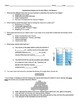# Mass, Volume, and Density AssessmentSubject
Resource Type
File Type

PDF

(330 KB|2 pages)
Product Rating
Standards
• Product Description
• StandardsNEW

Learning about the relationships between mass, volume, and density? Need to give an assessment on quantitative properties of matter?

This assessment covers finding mass and volume using measurement and water displacement. It also asks students to find density and use it to determine the identity of an object. This assessment can be used as a pre- and post-assessment at the beginning and end of a unit, as a formative assessment after a lab, or as a quiz at the end of a unit.

Create equations and inequalities in one variable and use them to solve problems.
Use ratio reasoning to convert measurement units; manipulate and transform units appropriately when multiplying or dividing quantities.
Apply the formulas 𝘝 = 𝘭 × 𝘸 × 𝘩 and 𝘝 = 𝘣 × 𝘩 for rectangular prisms to find volumes of right rectangular prisms with whole-number edge lengths in the context of solving real world and mathematical problems.
Recognize volume as an attribute of solid figures and understand concepts of volume measurement.
Convert among different-sized standard measurement units within a given measurement system (e.g., convert 5 cm to 0.05 m), and use these conversions in solving multi-step, real world problems.
Total Pages
2 pages
Included
Teaching Duration
N/A
Report this Resource to TpT
Reported resources will be reviewed by our team. Report this resource to let us know if this resource violates TpT’s content guidelines.